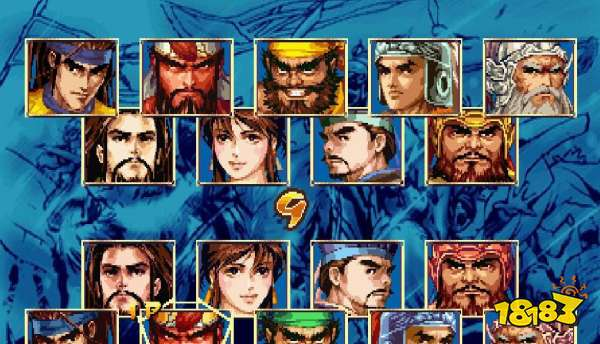# 三国战纪2群雄争霸出招表

【18183资讯】相信还有很多的小伙伴还不知道三国战纪2群雄争霸怎么出招，下面就由小编来告诉你们。

【18183资讯】相信还有很多的小伙伴还不知道三国战纪2群雄争霸怎么出招，下面就由小编来告诉你们。***所有降将的出招***

◎ →→(跑)

◎ A+B(去血保命招)

◎ B+C (抛弃道具)

◎ ↓+B(下蹲)

◎ →+B(突行)

◎ →+C(防)

◎ →+AAAAA(弹腿,可连打)

◎ ↓↘→+A(地堂斩)

◎ ↓↑+A(冲腿)

◎ 空中↓+AAAAA(空弹腿,可连打)

◎ 空中↓↘→+B(百鸟朝凤)

◎ ← →+B(兽咬)

◎ ↓↑+B(滚石阵)

◎ ↑↓+B(落石阵)

◎ ←↙↓↘→+B(火兽阵) 街机游戏下载中心 w w w.

◎ →→(跑)

◎ A+B(去血保命招)

◎ B+C (抛弃道具)

◎ ↓+B(下蹲)

◎ →+B(突行)

◎ →+C(防)

◎ ↓↑+A(大鹏展翅)

◎ 空中↓+A(探爪)

◎ 上式中+A(穿林脚)

◎ →+A (飞天,可连打)

◎ ↓↘→+B(日月双华)

◎ ← →+B (猛龙出闸)

◎ ↓↑+B(狂风术)

◎ ↑↓+B(长枪阵)

◎ ←↙↓↘→+B(火弓阵)

◎ →→(跑)

◎ A+B(去血保命招)

◎ B+C (抛弃道具)

◎ ↓+B(下蹲)

◎ →+B(突行)

◎ →+C(防)

◎ ↓↘→+A (迎风掸尘)

◎ ↓↘→+B(暴雪术)

◎ ← →+B(落雷术)

◎ ↓↑+B(暴风术)

◎ ↑↓+B(八阵图)

◎ ←↙↓↘→+B(恢复术) 街机游戏下载中心 www.66game.cn

◎ →→(跑)

◎ A+B(去血保命招)

◎ B+C (抛弃道具)

◎ ↓+B(下蹲)

◎ →+B(突行)

◎ →+C(防)

◎ 空中↓+A(饿虎扑羊)

◎ 突行中+A(饿虎张嘴)

◎ ↓↘→+A(狂风式)

◎ ↓↘→+B(神龙摆尾)

◎ ↓↑+B(火牛撼地)

◎ ↑↓+B(天崩地裂)

◎ ← →+B(混乱计)

◎ ←↙↓↘→+B(长枪阵) 街机游戏下载中心 www.66game.cn

◎ →→(跑)

◎ A+B(去血保命招)

◎ B+C (抛弃道具)

◎ ↓+B(下蹲)

◎ →+B(突行)

◎ →+C(防)

18183手游网发布此文仅为传递信息，不代表18183认同其观点或证实其描述。